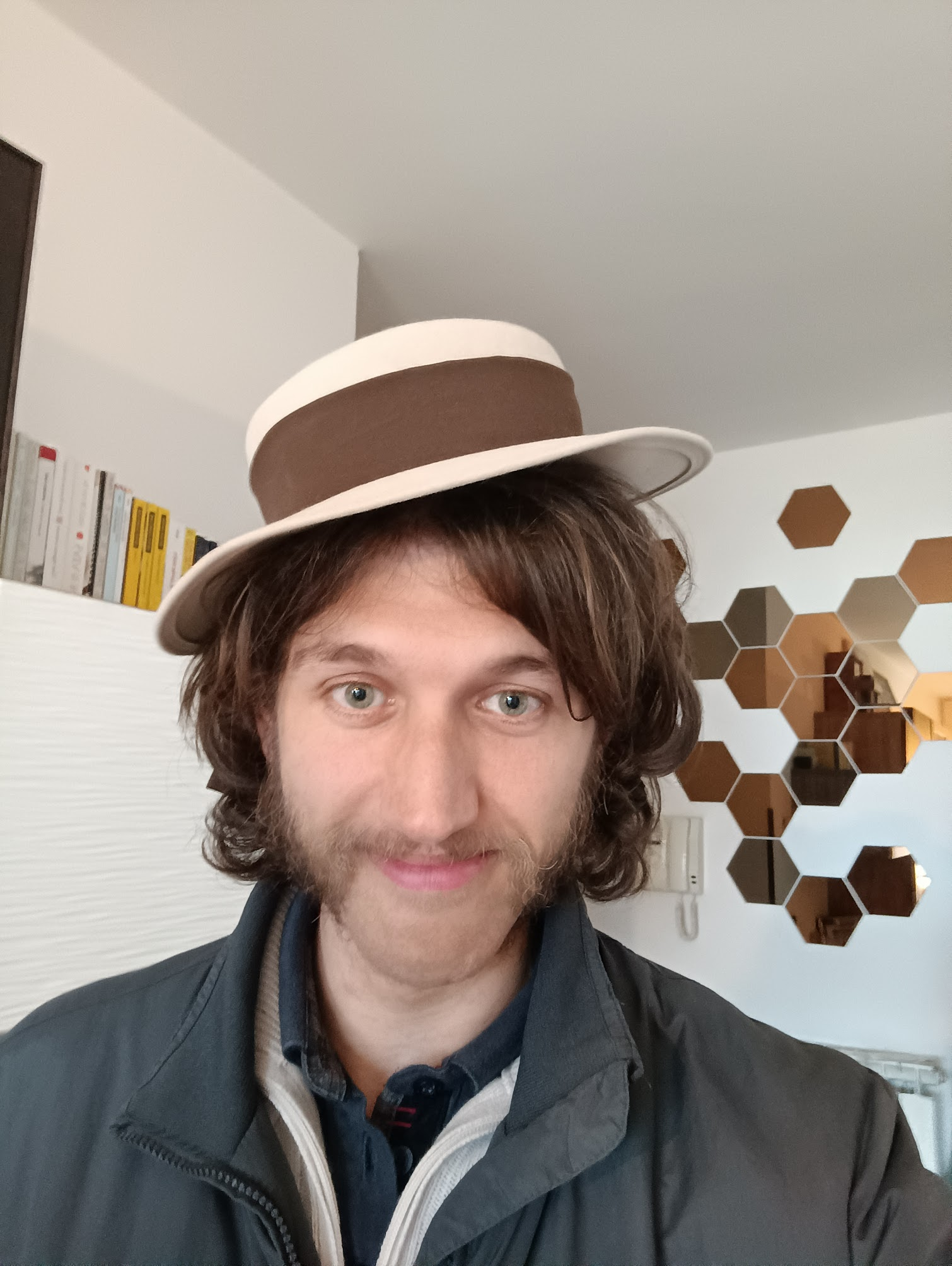# Davide Lombardo### Where to find me

Dipartimento di Matematica
Università di Pisa
Email: davide.lombardo@unipi.it
Office: 328 (first floor)
Telephone number: +39 050 2213297

### Research interests

The main focus of my research is the arithmetic of abelian varieties and the study of their associated Galois representations (in the spirit of the Mumford-Tate and Sato-Tate conjectures). This comprises questions ranging from pure arithmetic geometry (can we find a good description of the effect of base change on the Néron model of a non-semistable abelian variety?) to computational problems (how does one determine the endomorphism ring of the Jacobian of a nice curve over the rationals?)

### Some more specific questions

Below is a list of questions I would like to think about in the future. If you have ideas or comments about any of these, I'd love to hear them!

• Generalised Kummer theory. Instead of considering $\ell^n$-torsion points of abelian varieties (or more generally commutative algebraic groups), one can consider $\ell^n$-division points of a fixed rational point $\alpha \in A(K)$. Many natural problems can be cast in this setting, and while the situation is well understood when $\dim A=1$ and the ground field is $\mathbb{Q}$, other cases seem to be much more mysterious.

• Integral Galois theory. Let $L/K$ be a Galois extension of local or global fields with group $G$. What can be said about the structure of $\mathcal{O}_L$ as an $\mathcal{O}_K[G]$-module?

• Groups of components of Sato-Tate groups. The Sato-Tate conjecture is in a sense a refinement of the Mumford-Tate conjecture; Serre has shown how to attach a certain algebraic group $\operatorname{ST}(A/K)$ to an Abelian variety $A$ over a number field $K$, and the group of components $\operatorname{ST}(A/K) / \operatorname{ST}(A/K)^0$ is thought to have significant arithmetical interest. There are many questions that can be asked about this object, for example: there are some 'obvious' constraints on which finite groups can be component groups of Sato-Tate groups over a given field $K$. Are these conditions sufficient?

• Geometric realisation of endomorphisms of Jacobians. Every endomorphism of $\operatorname{Jac}(C)$, where $C$ is a smooth projective curve, can be represented by a divisor on $C \times C$. In some situations, these divisors have 'conceptual' interpretations, and I would like to (identify and) explore more of these cases.

• Local-global principles for isogenies of abelian varieties.

• Integral Lie theory. A wonderful result by Richard Pink gives a very handy description of the pro-$p$ subgroups of $\operatorname{SL}_2(\mathbb{Z}_p)$ for $p>2$. I have managed to partially extend this to also cover the case $p=2$, but no similar result is available for algebraic groups other than $\operatorname{SL}_2$. Lazard's theory of Lie algebras should be relevant here, but work remains to be done.

• The inverse problem for automorphism groups. Let $G$ be an algebraic group. Is there a projective variety $X$ with $\operatorname{Aut}(X) \cong G?$ We now know the answer when $G$ is an abelian variety, and I would love to understand whether certain linear algebraic groups are automorphism groups of smooth varieties or not.

• Rational points of moduli stacks of curves. It is well known that elliptic curves over an algebraically closed field are classified by their $j$-invariant. Elliptic curves $E$ over a non-algebraically closed field $K$ of characteristic $\neq 2,3$ are classified by $j(E)$ together with the datum of a class in $K^\times/K^{\times d}$, where $d = \# \operatorname{Aut}(E_{\overline{K}})$. Can a similar description be found for genus 2 curves -- or, more interestingly, can one show that no such description exists (under suitable 'functoriality' assumptions)?